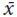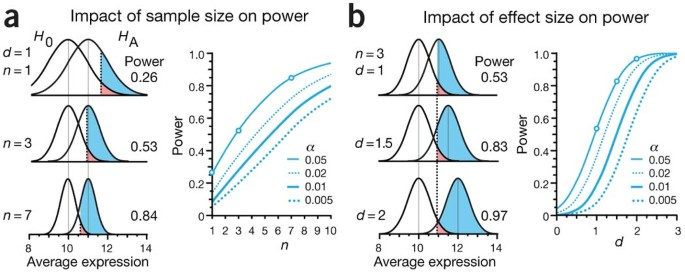Statistical testing provides a paradigm for deciding whether the data are or are not typical of the values expected when the hypothesis is true. Because our objective is usually to detect a departure from the null hypothesis, it is useful to define an alternative hypothesis that expresses the distribution of observations when the null is false. The difference between the distributions captures the experimental effect, and the probability of detecting the effect is the statistical power.

Statistical power is critically relevant but often overlooked. When power is low, important effects may not be detected, and in experiments with many conditions and outcomes, such as 'omics' studies, a large percentage of the significant results may be wrong. Figure 1 illustrates this by showing the proportion of inference outcomes in two sets of experiments. In the first set, we optimistically assume that hypotheses have been screened, and 50% have a chance for an effect (Fig. 1a). If they are tested at a power of 0.2, identified as the median in a recent review of neuroscience literature1, then 80% of true positive results will be missed, and 20% of positive results will be wrong (positive predictive value, PPV = 0.80), assuming testing was done at the 5% level (Fig. 1b).Figure 1: When unlikely hypotheses are tested, most positive results of underpowered studies can be wrong.

In experiments with multiple outcomes (e.g., gene expression studies), it is not unusual for fewer than 10% of the outcomes to have an a priori chance of an effect. If 90% of hypotheses are null (Fig. 1a), the situation at a 0.2 power level is bleak—over two-thirds of the positive results are wrong (PPV = 0.31; Fig. 1b). Even at the conventionally acceptable minimum power of 0.8, more than one-third of positive results are wrong (PPV = 0.64) because although we detect a greater fraction of the true effects (8 out of 10), we declare a larger absolute number of false positives (4.5 out of 90 nulls).

Fiscal constraints on experimental design, together with a commonplace lack of statistical rigor, contribute to many underpowered studies with spurious reports of both false positive and false negative effects. The consequences of low power are particularly dire in the search for high-impact results, when the researcher may be willing to pursue low-likelihood hypotheses for a groundbreaking discovery (Fig. 1). One analysis of the medical research literature found that only 36% of the experiments examined that had negative results could detect a 50% relative difference at least 80% of the time2. More recent reviews of the literature1,3 also report that most studies are underpowered. Reduced power and an increased number of false negatives is particularly common in omics studies, which test at very small significance levels to reduce the large number of false positives.

Studies with inadequate power are a waste of research resources and arguably unethical when subjects are exposed to potentially harmful or inferior experimental conditions. Addressing this shortcoming is a priority—the Nature Publishing Group checklist for statistics and methods (http://www.nature.com/authors/policies/checklist.pdf) includes as the first question: “How was the sample size chosen to ensure adequate power to detect a pre-specified effect size?” Here we discuss inference errors and power to help you answer this question. We'll focus on how the sensitivity and specificity of an experiment can be balanced (and kept high) and how increasing sample size can help achieve sufficient power.

Let's use the example from last month of measuring a protein's expression level x against an assumed reference level μ0. We developed the idea of a null distribution, H0, and said that x was statistically significantly larger than the reference if it exceeded some critical value x* (Fig. 2a). If such a value is observed, we reject H0 as the candidate model.

Because H0 extends beyond x*, it is possible to falsely reject H0, with a probability of α (Fig. 2a). This is a type I error and corresponds to a false positive—that is, inferring an effect when there is actually none. In good experimental design, a is controlled and set low, traditionally at α = 0.05, to maintain a high specificity (1 − α), which is the chance of a true negative—that is, correctly inferring that no effect exists.

Let's suppose that x > x*, leading us to reject H0. We may have found something interesting. If x is not drawn from H0, what distribution does it come from? We can postulate an alternative hypothesis that characterizes an alternative distribution, HA, for the observation. For example, if we expect expression values to be larger by 20%, HA would have the same shape as H0 but a mean of μA = 12 instead of μ0 = 10 (Fig. 2b). Intuitively, if both of these distributions have similar means, we anticipate that it will be more difficult to reliably distinguish between them. This difference between the distributions is typically expressed by the difference in their means, in units of their s.d., σ. This measure, given by d = (μAμ0)/σ, is called the effect size. Sometimes effect size is combined with sample size as the noncentrality parameter, dn.

In the context of these distributions, power (sensitivity) is defined as the chance of appropriately rejecting H0 if the data are drawn from HA. It is calculated from the area of HA in the H0 rejection region (Fig. 2b). Power is related by 1 − β to the type II error rate, β, which is the chance of a false negative (not rejecting H0 when data are drawn from HA).

A test should ideally be both specific (low false positive rate, α) and sensitive (low false negative rate, β). The α and β rates are inversely related: decreasing α increases β and reduces power (Fig. 2c). Typically, α < β because the consequences of false positive inference (in an extreme case, a retracted paper) are more serious than those of false negative inference (a missed opportunity to publish). But the balance between α and β depends on the objectives: if false positives are subject to another round of testing but false negatives are discarded, β should be kept low.

Let's return to our protein expression example and see how the magnitudes of these two errors are related. If we set α = 0.05 and assume normal H0 with σ = 1, then we reject H0 when x > 11.64 (Fig. 3a). The fraction of HA beyond this cutoff region is the power (0.64). We can increase power by decreasing specificity. Increasing α to 0.12 lowers the cutoff to x > 11.17, and power is now 0.80. This 25% increase in power has come at a cost: we are now more than twice as likely to make a false positive claim (α = 0.12 vs. 0.05).

Figure 3b shows the relationship between α and power for our single expression measurement as a function of the position of H0 rejection cutoff, x*. The S-shape of the power curve reflects the rate of change of the area under HA beyond x*. The close coupling between a and power suggests that for μA = 12 the highest power we can achieve for α ≤ 0.05 is 0.64. How can we improve our chance to detect increased expression from HA (increase power) without compromising α (increasing false positives)?

If the distributions in Figure 3a were narrower, their overlap would be reduced, a greater fraction of HA would lie beyond the x* cutoff and power would be improved. We can't do much about σ, although we could attempt to lower it by reducing measurement error. A more direct way, however, is to take multiple samples. Now, instead of using single expression values, we formulate null and alternative distributions using the average expression value from a samplethat has spread σ/√n (ref. 4).

Figure 4a shows the effect of sample size on power using distributions of the sample mean under H0 and HA. As n is increased, the H0 rejection cutoff is decreased in proportion with the s.e.m., reducing the overlap between the distributions. Sample size substantially affects power in our example. If we average seven measurements (n = 7), we are able to detect a 10% increase in expression levels (μA = 11, d = 1) 84% of the time with α = 0.05. By varying n we can achieve a desired combination of power and α for a given effect size, d. For example, for d = 1, a sample size of n = 22 achieves a power of 0.99 for α = 0.01.Figure 4: Impact of sample (n) and effect size (d) on power.

Another way to increase power is to increase the size of the effect we want to reliably detect. We might be able to induce a larger effect size with a more extreme experimental treatment. As d is increased, so is power because the overlap between the two distributions is decreased (Fig. 4b). For example, for α = 0.05 and n = 3, we can detect μA = 11, 11.5 and 12 (10%, 15% and 20% relative increase; d = 1, 1.5 and 2) with a power of 0.53, 0.83 and 0.97, respectively. These calculations are idealized because the exact shapes of H0 and HA were assumed known. In practice, because we estimate population σ from the samples, power is decreased and we need a slightly larger sample size to achieve the desired power.

Balancing sample size, effect size and power is critical to good study design. We begin by setting the values of type I error (α) and power (1 − β) to be statistically adequate: traditionally 0.05 and 0.80, respectively. We then determine n on the basis of the smallest effect we wish to measure. If the required sample size is too large, we may need to reassess our objectives or more tightly control the experimental conditions to reduce the variance. Use the interactive graphs in Supplementary Table 1 to explore power calculations.

When the power is low, only large effects can be detected, and negative results cannot be reliably interpreted. Ensuring that sample sizes are large enough to detect the effects of interest is an essential part of study design.